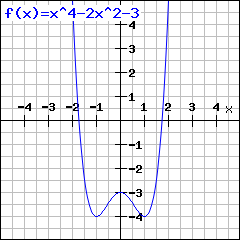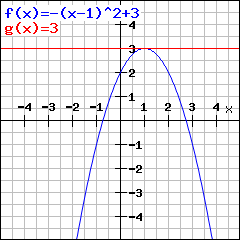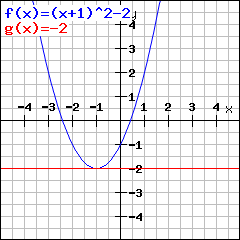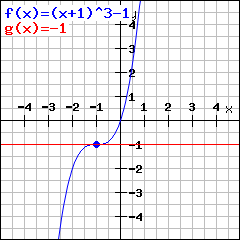# Calculate turning pointsEnter the function whose turning points you want to calculate. Hint: Enteras 3*x^2 ,as 3/5 andas (x+1)/(x-2x^4) To write powers, use ^. This means, you gotta write x^2 for.

## What is a turning point?

A turning point is a point where the graph of a function has the locally highest value (called a maximum turning point) or the locally lowest value (called a minimum turning point). A function does not have to have their highest and lowest values in turning points, though.This graph e.g. has a maximum turning point at (0|-3) while the function has higher values e.g. in (2|5). This implies that a maximum turning point is not the highest value of the function, but just locally the highest, i.e. there is no higher value at least in a small area around that point.

## How to find turning points?

The basic idea is that tangents in a turning point have slope.So the basic idea of finding turning points is:
• Find a way to calculate slopes of tangents (possible by differentiation).
• Find when the tangent slope is. There could be a turning point (but there is not necessarily one!)

This means: To find turning points, look for roots of the derivation.

## Does slopealways imply we have a turning point?

No. If the slope is, we max have a maximum turning point (shown above)or a mininum turning pointor the slope just becomesfor a moment though you have no turning point. Such a point is called saddle point.

## Does the slope always have to bein turning points?

Yes. This is right. But not the reversion, as seen above. So we say: Having slopeis necessary but not sufficient for having a turning point.

## Let's assume we have slope. How can I find out if I have a maximum / minimum turning point or a saddle point?

By using the change of signs criterion.

## I have to find turning points as homework and don't know how. What can I do?

Just enter your function above. Mathepower does the calculation, with explanation and step-by-step.